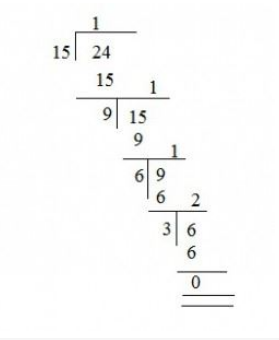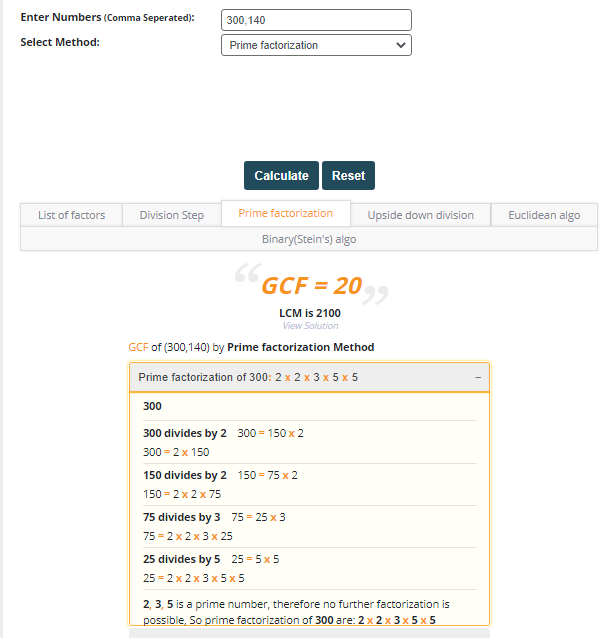# What are the best ways to find highest common factor?Almost every student who has studied Mathematics must be familiar with HCF, The Highest Common Factor.

This article defines, what are the best ways to find the highest common factor?

But before going deeper into it, we need to first understand what a Highest common factor is?

This will be explained below along with a few examples to make you understand better.

# What is a Highest Common Factor?

The Highest Common factor, also known as HCF, of 2 numbers is the largest number that can evenly divide any given 2 numbers and is common in both.

Don’t get confused, if anybody encounters other names of HCF, they all have the same meaning, the alternate names of HCF are:

• Greatest common divisor (GCD)
• Greatest common factor (GCF)

For example:

The highest or greatest common factor in between 2 random number like 4 and 16 is 4

While defining HCF according to arithmetic standards, it’s the largest common divisor or GCF of 2 or more (+ve) integers, this occurs the largest (+ve) integer and dividing the random figures without leaving any value or remainder.

## Best ways to find highest common factor

### Euclid’s Algorithm

Sometimes people have to find GCF of very large numbers like 387444,239001 and 162001, this is where Euclid’s method is usually used to find out the Highest common factor.

According to Euclid’s method, it has been stated that the best way is to:

First of all, subtract the smaller number from the larger number given two whole numbers and then see the result.

Again the process is repeated until you get a smaller number than the first result.

Takedown the first smallest number as the new highest number and then subtract the result from the new bigger number which was driven out in the second phase.

### Play around methodology

This another best way to find out HCF, Usually teachers practice it while teaching kids in schools.

It’s like you keep on playing with the numbers until you get the common factor.

For instance

In this example let’s take two number as 6 and 24

6 x 1=6, 6 x 4 =24

Well in this case the highest common factor is 6

### Factoring methodology

There are two ways to perform this

One can be done by using a factorizing calculator

Secondly through finding out all the factors or every number.

For example

A number such as 16 has 5 factors these are 1, 2, 4, 8, and 16

A number such as 24 has 8 factors these are 1, 2, 3, 4, 6, 8, 12, and 24

A number such as 32 also has 8 factors these are 1, 2, 4, 8, 16, and 32

Looking at these factors, it’s clear that the greatest common factor of 16, 24, and 32 is (1, 2, 4, and 8)

### Division methodology

To perform this method you need 2 of the given numbers for finding HCF.

First, start by dividing the larger number by a smaller number and then the divisor by the remainder.

Now again divide the divisor of this division again by the next remaining that has been found and keep on repeating until the remainder becomes zero.

For example

In this example, we need to find HCF of 15 and 24 so let’s see how it works

See also  Mastering the States and Capitals of India: A Comprehensive Guide

So through this method, we can see the HCF of 15 and 24 is 3.### Online Calculators

These GCF finder online calculators are specifically designed for students, teachers, and common people to easily find the greatest common factor or HCF.

Nowadays as everyone is a technology lover, these specific educational online tools are a great addition to our communities as they can help anyone find out instantly without doing any longer mathematics.

For example

Meracalculator is a great example to find out quick and accurate results.

Let’s see how it works

• Step1- Visit https://www.meracalculator.com/math/gcf.php
• Step2- Enter the values like 300, 140
• Step3-Select any method you like from division, prime factor, Euclidian, binary, upside down, and list of factors
• Step 4- Click the option calculate
• Step 5- It will show you the GCF = 20From all the different ways of finding HCF, using an online calculator seems to be the best and time-saving.

### Prime factors

Finding out a prime number is quite simple because primary factors are termed as the factors of a number which are themselves prime numbers.

Usually using this method is like creating a tree of prime factors or you can also get the prime factor by combing the common ones.

For example

In this example, we are going to take 36 and 120

The prime factor of 36 are 2 x 2 x 3x 3 =36

The prime factor of 120 are 2 x 2 x 2 x 3 x 5 = 120

So we found out the HCF of 36 and 120 = 12

### Why does HCF matter?

• One of the greatest benefits of HCF is when people are looking to simplify a fraction
• Distribute things equally in a proper portion
• To arrange or organize things equally
• In our daily mathematic equations
##### Conclusion

Anyone looking to easily find out an HCF or GCF of two or more values can use any of the above mentioned best ways to quickly get the desired results.